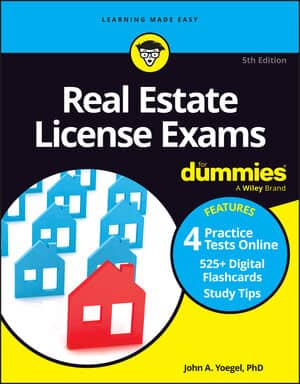##### Real Estate License Exams For DummiesThere will be questions on the Real Estate License Exam about the GRM technique. The Gross Rent Multiplier (GRM) technique for estimating value is based on the idea that a property value can be calculated as a multiple of the gross rent. The formula states this succinctly:

Gross rent x GRM (factor) = value estimate

The gross rent is the monthly income of the building with no deductions for expenses. Another rent factor is called the gross income multiplier (GIM). In this case, the income used is gross annual rent rather than monthly. Other than that, all the formulas and resulting values are the same.

You’re now correctly asking, “So where do I get the GRM factor?” As with all the data appraisers use, they calculate it based on the market. After getting all the information needed on the subject property, the appraiser researches the market for that type of property, which is usually the local market, to obtain a number of comparable sales.

Comparable sales are recent sales of similar buildings. After locating a number of similar buildings, the appraiser needs to find the sales price and the gross income of each building. By dividing the sales price of the building by the gross rent, the appraiser obtains a gross rent multiplier for each of the comparables. If the buildings are similar, the gross rent multipliers also will be similar numbers.

Say you’re appraising a three-unit residential rental property. The gross monthly rent of this property is \$600 per apartment or \$1,800 per month for the whole building. You research similar buildings and find three comparable properties that are in the same location, approximately the same size, and that generate approximately the same gross monthly rent. The data is shown here:

Sale price Gross monthly rent
\$200,000 \$2,000
\$180,000 \$1,800
\$190,000 \$1,900

You now apply the formula for finding the GRM to this data.

Sales price ÷gross monthly rent = GRM

Applying the formula to the example data, you find that the GRM for each of the comparables would be 100, which means that the comparable buildings each sold for a price that was 100 times its gross monthly rent. The final step is to apply the value formula to the subject property:

Gross monthly rent x GRM = value estimate

\$1,800 x 100 = \$180,000

The GRM method is particularly useful for small-income-producing properties, such as one-to-four-family houses.